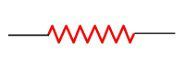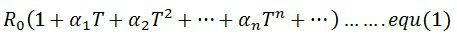# Difference Between RTD & Thermistor

The Thermistor and the RTD both are the temperatures measuring device. The major difference between the RTD and the Thermistor is that the RTD is made of metal, whereas the semiconductor material is used for Thermistor.  The other differences between the Thermistor and RTD are explained below in the comparison chart.

As the Thermistor is made of semiconductor material, they have both the negative and positive temperature coefficient. But most of them thermistor have a negative temperature coefficient, i.e., their resistance decreases with the increases in temperature. The negative temperature coefficient can be large enough for several percentage increases in temperature because of the Thermistor can detect the small changes in temperature.

The RTD is made of pure metal like platinum, nickel, etc., which has a positive temperature coefficient. The positive temperature coefficient means their resistance increases with the temperature, which is the main function of RTD. The RTD is used for measuring the large variation in temperature.

## Comparison Chart

Basis For Comparison RTD (Resistance Temperature Detector)Thermistor
Definition The device use for measuring the change in temperature is known as the RTD or Resistance Temperature Detector.It is a thermal resistor whose resistance changes with the temperature.
SymbolMaterialMetals (platinum, nickel, copper, etc.)Semiconductor
Accuracy Less accurate. Their accuracy is high. It can detect even small changes in temperature because of negative temperature coefficient.
Response Time Slow Fast
Temperature Range Up to 850ºC-55°C and + 114°C
Characteristic GraphLinear Non-linear
Sensitivity Low High
SizeLarge Small
Cost CheapExpensive
Resistivity High Low
Hysteresis Effect LowHigh
Applications In industries for measuring large temperature. For measuring the temperature of home appliances.

### Definition of RTD

The RTD stands for Resistance Temperature Detector. The RTD is defined as the resistor which is used for measuring the temperature. It works on the principle that the resistance changes with the change in temperature.The resistance of the conductor change with temperature and this property of conductors is used in the RTD for measuring the temperature. The RTD is made of pure metals like platinum, nickel, copper etc. The change of resistance concerning with the conductor is explained by the equation shown below.The platinum is used in RTD because it can withstand high temperature and also it has high stability. The metals have the high-temperature coefficient, i.e. their temperature increases with the increases in temperature and this property of metals are used in the RTD.

### Definition of Thermistor

The thermistor is a thermal resistor used for measuring temperature.  It is made of semiconductor material because of which it has both the positive and negative temperature coefficient. The positive temperature coefficient means temperature increases with the increases in resistance and in negative temperature coefficient the temperature is inversely proportional to the resistance.The thermistor is used for measuring the small range of temperature, which is nearly up to -60ºC – 15ºC. The range of resistor in the thermistor is from 0.5 – 0.75 Ω. It is an extremely sensitive device used for measuring the temperature of home appliances.

## Key Differences Between RTD and Thermistor

The following are the key differences between the RTD and the Thermistor.

1. The RTD is a type of instrument used for measuring the temperature, whereas, the thermistor is the thermal resistor whose resistance changes with temperature.
2. The RTD is made of the metals having a positive temperature coefficient whereas the thermistor is made of the semiconductor materials.
3. The accuracy of the RTD is low as compared to the thermistor. The thermistor has a negative temperature coefficient because of which they can detect even the smallest change in temperature.
4. The thermistor gives a quick response to the small changes, whereas the response time of the RTD is slow.
5. The thermistor is used for measuring the small range of temperature (between -55°C and + 114°C) whereas the RTD measures the temperature up to 850ºC.
6. The characteristic graph between resistance and temperature of the RTD is linear whereas that of thermistor it is nonlinear.
7. The RTD is less sensitive as compared to the thermistor.
8. The size of the RTD is much larger as compared to the thermistor.
9. The cost of the thermistor is much higher than the RTD.
10. The resistivity of the RTD is less as compared to the thermistor.
11. The hysteresis effect in the thermistor is much high as compared to the RTD.
12. The RTD is used in the industries for measuring the high temperature, whereas the thermistor is used in home appliances for measuring the small temperature.

### Conclusion

The RTD and thermistor both are the temperature sensing device. The RTD is used for measuring the high temperature, and the thermistor is used for measuring the temperature of small devices.